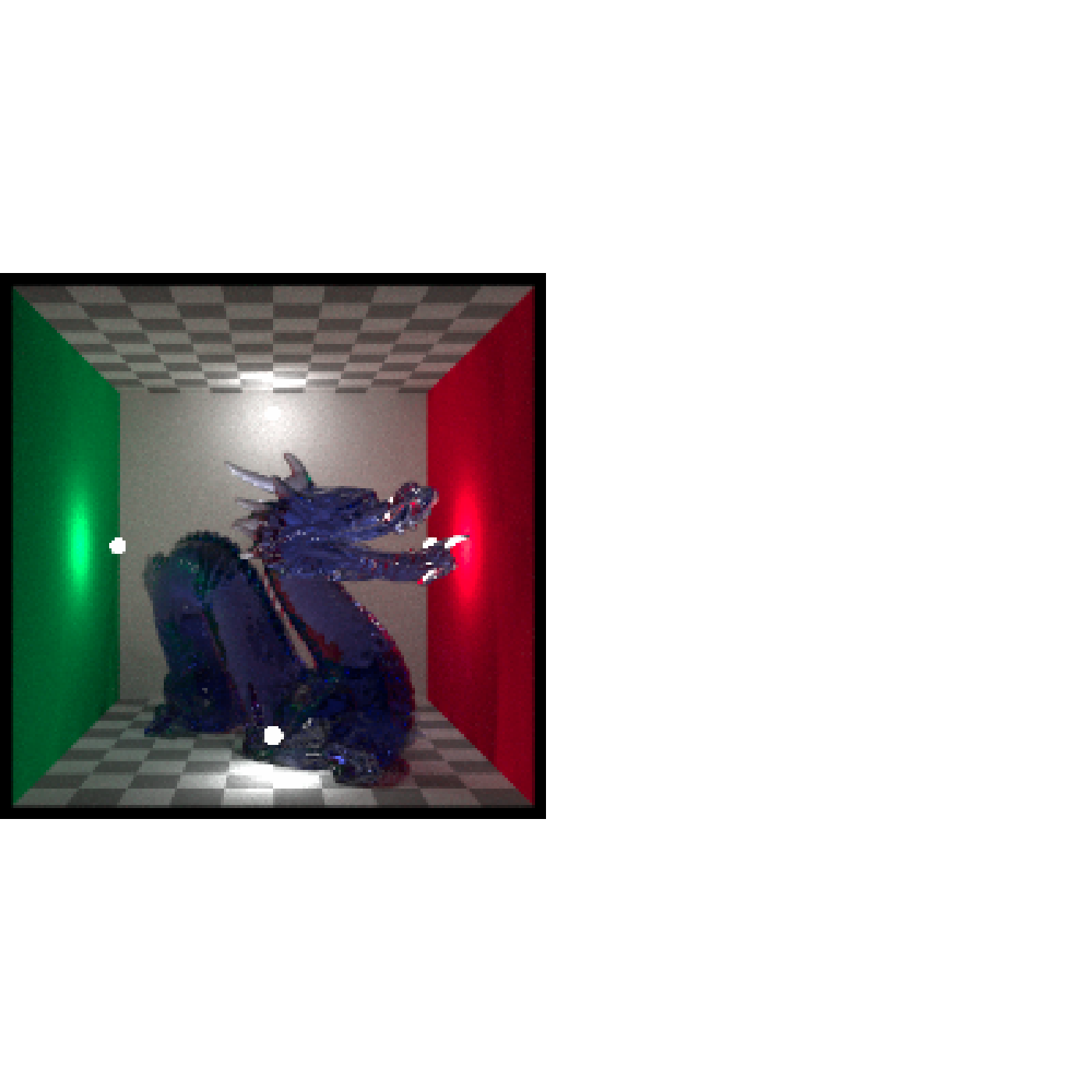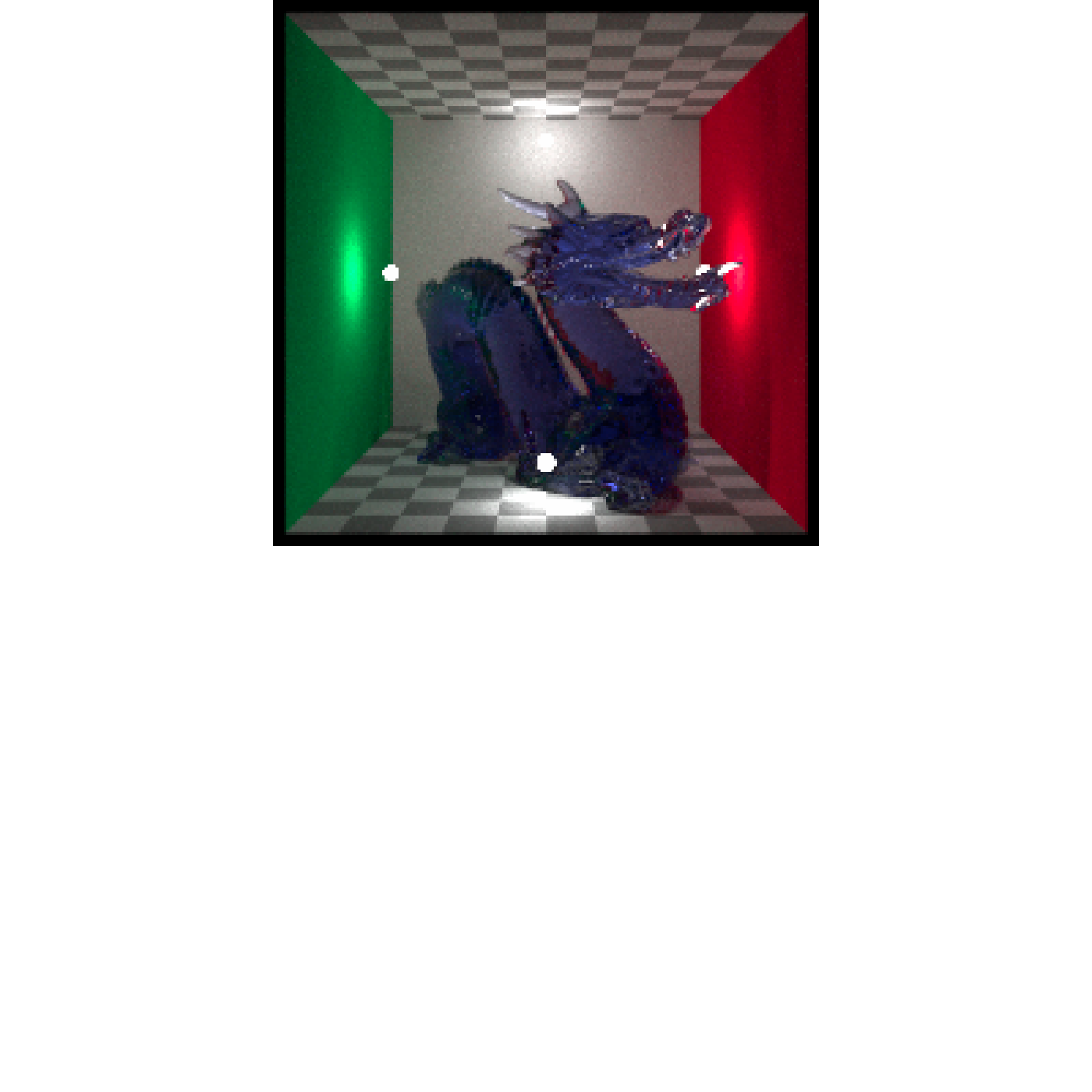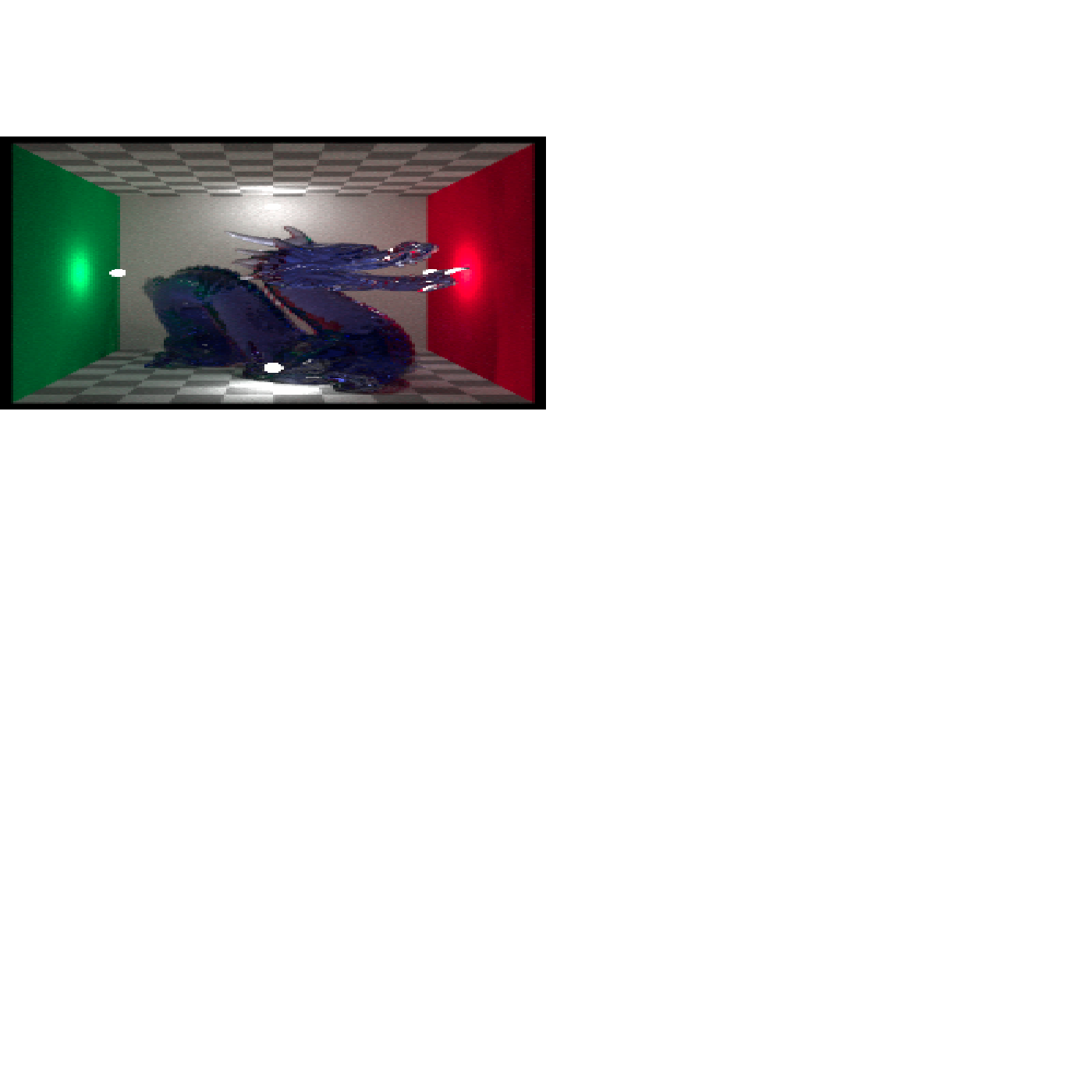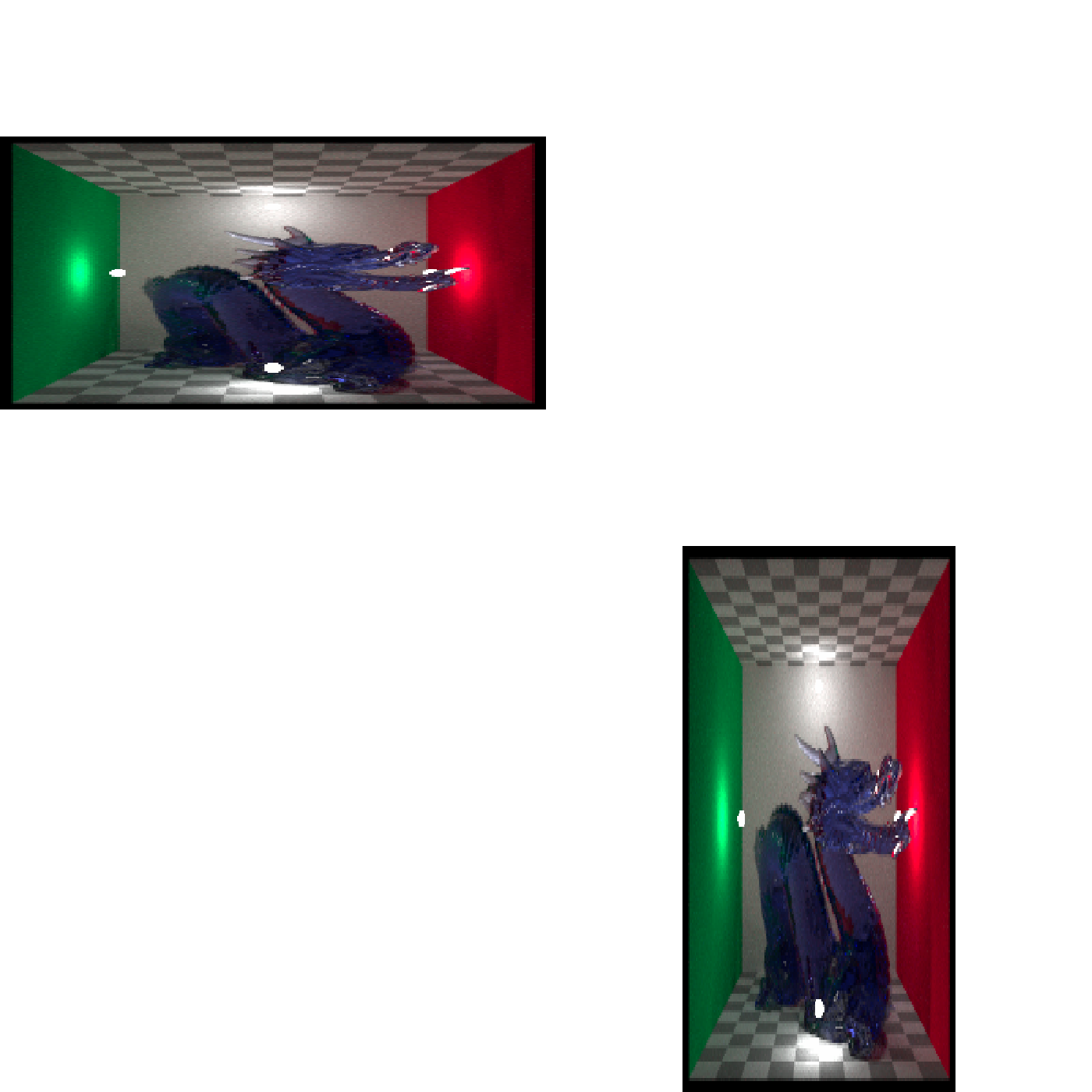Displays the image in the current device.

plot_image_grid(input_list, dim = c(1, 1))

## Arguments

input_list

List of array (or matrix) image inputs.

dim

Default c(1,1). Width by height of output grid.

## Examples

if(rayimage:::run_documentation()){
#Plot the dragon array
plot_image_grid(list(dragon, dragon), dim = c(1,2))
}if(rayimage:::run_documentation()){
plot_image_grid(list(dragon, dragon), dim = c(2,1))
}if(rayimage:::run_documentation()){
plot_image_grid(list(dragon, dragon), dim = c(2,2))
}if(rayimage:::run_documentation()){
#Plot alongside the depth matrix
dragon_depth_reoriented = render_reorient(dragondepth,
transpose = TRUE,
flipx = TRUE)/2000
plot_image_grid(list(dragondepth/2000, dragon, dragon, dragondepth/2000),
dim = c(2,2))
}